## Predictor tool in moodss

### Contents:

This document contains general and reference information to help the user understand how to use the predictor tool, and the statistical methods used in its implementation.

### 2. Introduction

Using sophisticated statistical techniques and artificial neural networks, it is possible, using a powerful computing platform, to predict the future behavior of data, based on its history.
The predictor tool first displays the existing history of a data cell, from the moodss database. Using the predictor controls, the user prepares the data for analysis, then lets the computer find the best statistical model that describes, or rather fits, the existing data, model which is finally used to predict the future values of the data, within a reasonable time frame.

### 3. Example

Let us start with a simple example as an introduction to the full capabilities of the tool.

First make sure that sample time series are included in the database, from the Preferences Tools/Predictor section.
Next, using the File/Database/Load menu, expand and select the instance of the AirPassengers module from the database instances dialog box. Then, using the Tools/Predictor menu, create a new predictor and drag and drop the AirPassengers value cell into it. You should see a graph as shown in the following picture.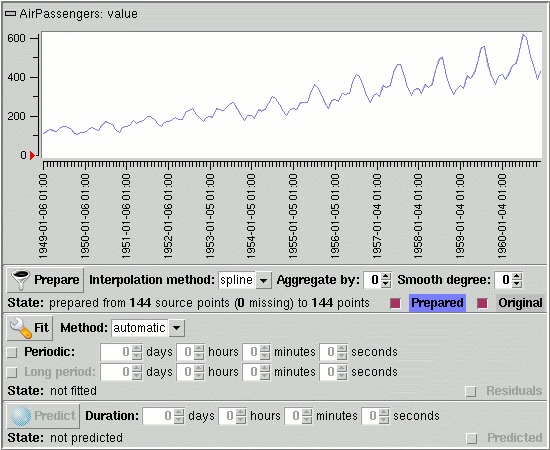Next, click on the Prepare button to obtain "clean" data with equally spaced time samples. After a short while, the Prepared label should light up and the prepared data graph should cover the original data graph. Take this opportunity to change the colors of the Original and prepared graphs by using the colors popup menus from a mouse right button click on the corresponding labels.
You should then obtain a predictor tool in a state similar to the picture above.

Next, let the computer find the best statistical model for the prepared data.
You must have noticed, by looking at the graph, that even if the number of passengers globally increases with time, every year, it roughly behaves as in the previous year, with a peak during the summer season. Consequently, let the optimizing algorithms know that the data has a period of 1 year by enabling the Periodic entry row and inputting a period of 365 days.
Note: also try and let the statistical engine estimate the main period by clicking on the button on the right side of the periodic row.

Click on the Fit button. The computer should then try many different models using the ARIMA then neural techniques, as the following picture shows.
Note: this takes more than 1 hour on a Pentium 4 2.4 GHz machine.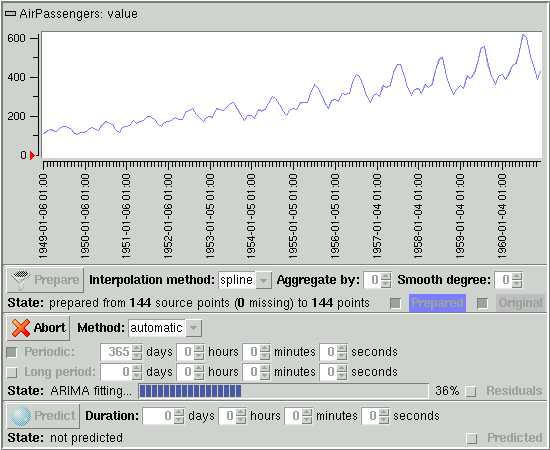The following picture shows the result of the fitting process, with an ARIMA model, its parameters enclosed in parentheses, found as best model. The Residuals graph has become visible at the bottom of the plot area, with reasonably small values (the smaller the fitting errors the better the fit of course).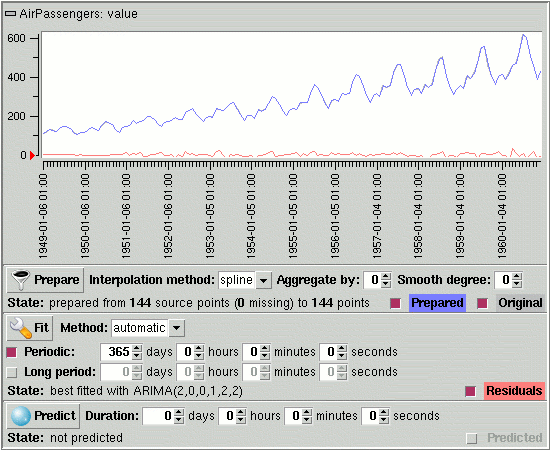Since we know have a good model on a 12 year history, predicting the data behavior over 1 year seems reasonable. After inputting 365 days as duration and hitting the Predict button, the result of the completed process is displayed, as the following picture shows.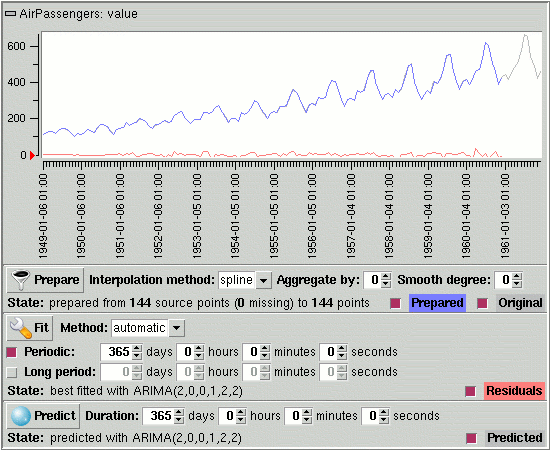You may have noticed, during the preceding operations, that the trace window popped up (see picture below). It may happen when the statistical calculation engine reports warnings or errors when processing the data. In this case, you may safely ignore the warning.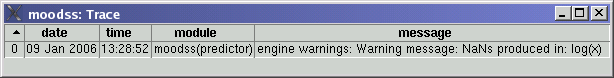### 4. Manual

Predicting data behavior in the future consists in finding a reproducible way, statistical or via an artificial neural network, to describe the behavior of the data history from its past to the current time. Once this optimal model is known, it becomes quite simple to predict the future values of the data being studied, within a reasonable duration of course, like around 10 percent of the history range.
This is called time series analysis. In order for the analysis to be properly performed, the data must first be transformed into a regular time series. This operation occurs in the preparation stage, using a process called interpolation. Preparing may also include an aggregation stage if there are so many data samples that too much processing would be required to find the data model. The data may also be smoothed at this point to remove small variations with little consequence on the global behavior of the data in its history.
Once the data is prepared and ready, comes the lengthy part of the analysis: finding the statistical or neural model that best fits the studied data. This is handled by the computer which will try many combinations, which can take from minutes up to a few days, depending on the speed and memory resources of your computing environment. This is when you need a fast multi-processor machine...
Finally, predicting the data is the closing stage of the process, a reward to be taken with reasonable doubt, as it seems the future cannot yet be reliably predicted (or else you would not be reading this...).

The actual calculations are handled by R processes, R being the powerful statistical computing engine and environment (at www.r-project.org).
A powerful computing platform is needed to perform statistical computations: a 1 GHz processor with 512 megabytes of memory is a minimum and a multi-processor 2 GHz machine with 1 gigabytes of memory usable (the more processors the better).

Finally, I strongly encourage the user to practice and improve using the sample time series included in the database (see Preferences Tools/Predictor section on how to enable them) before attempting to perform predictions...

#### 4.1. Preparing data

In order to be able to find the best statistical model describing the behavior of the data, the history of which comes from the moodss database, the cell data must be transformed into a regular time series, with samples equally spaced in time and no missing values, a reasonable enough number of samples so that fitting calculations do not take too long, and rough variations smoothed out.

The first mandatory step is called interpolation, which is a method of constructing new data points from a discrete set of known data points. Once the data cell has been dropped into the tool, and its time range set using the View/Database range menu, choose an Interpolation method:

• spline: preferred method, where constructed points lie on smooth curves going through the original data points. Nevertheless, non optimal if big holes occur in the original data, as the following picture shows (AirPassengers data with an artificially created void filled with spline in blue)
• linear: method where constructed points simply lie on straight line segments, and which behaves better in some cases (see picture below with void linearly filled in red)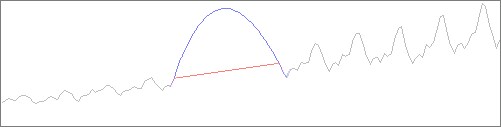In order to diminish the computing time needed for the following fitting stage, it is advised to reduce the number of data points that the statistical engine will have to work on, while preserving the aspect of the original curve. This process is called aggregation, where a number of adjacent points are combined into a single data point, whose value is the mean of the original values. For example, as shown in the following picture, we chose to Aggregate by 10, thereby dividing the number of data points to process by 10 (and the fitting time by about 6):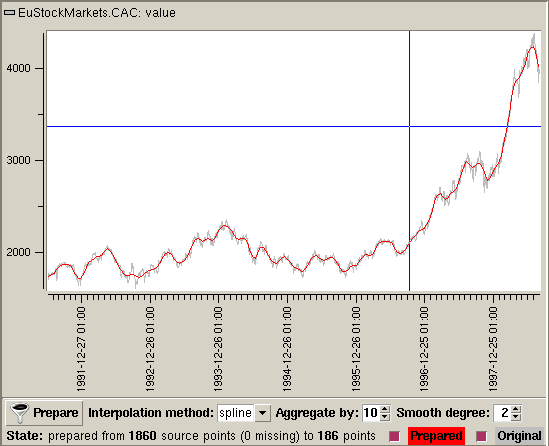Finally, ignoring small variations in the data also improves the fitting time and the precision of predictions. This is accomplished by setting the Smooth degree, as shown in the preceding picture, where it was set to 2.
As an experiment, you may also try increasing the smoothing degree to the point where you can visualize the data trend.

Once all the preparation parameters are set, click on the Prepare button. After a short while, the Prepared label lights up and the prepared data graph covers the original data graph. Note that you can change the colors of the Original and prepared graphs by using the colors popup menus from a mouse right button click on the corresponding labels. You can also display or hide any graph by clicking on check buttons next to the colored labels.

After some iterations of the above processes, the data is now ready for the next stage.
It is obvious that the fitting of the statistical model, and therefore the predictions, will be based on the prepared data, not the original one. It is therefore very important that the data behavior be known by the user, who will then be able to find the best compromise between precision of the prepared data and computing resources needed to find the best statistical model.

#### 4.2. Fitting process

Fitting consists in finding the model that best describes the behavior of the prepared data. The predictor tool supports 2 Methods to achieve that goal:

• ARIMA: AutoRegressive Integrated Moving Average, a powerful and flexible statistical model with an optional seasonal effect in order to better handle periodic data
• neural: artificial neural network, an interconnected group of artificial neurons, which has the ability to "learn" from the data and is flexible enough to handle bi-periodic data
Note that the automatic method choice results in the tool picking the best of the best ARIMA and best neural models found.
Note: for detailed information on the actual techniques used, see the statistics techniques section below.

You should know whether the data is periodic or not, based on your experience and the aspect of the original data graph. For computer related data, it is often a 1 day period as users come to work every day, start working in the morning and leave at night, using computers, network, databases, ... during their presence most of the time. The special case of low activity on week-ends is examined in an example below.
Note that you can get help from the statistical engine to estimate the main period by clicking at any time on the button on the right side of the Periodic row. If, using a fast Fourier transform (FFT), the period can be calculated, it replaces the current periodic duration, which may then be manually adjusted to the nearest number of days, for example.

The ARIMA method can handle periodic data but with a single period only. If you select the ARIMA method, only the Periodic duration entry row is enabled. Click on the corresponding check button and input the data period. Note that you may leave the duration at 0 if you suspect that the data is periodic but you do not know its period: the ARIMA algorithm will try both non-periodic and periodic models and pick the best.
Note that the calculations take a much longer time when the data is specified as periodic (more than 30 times as much for the AirPassengers data when period is set to 0 and more than 150 times as much when period is set to 365 days! (which nevertheless results in a better fit)).

The neural method can handle periodic data with 1 or 2 periods, but cannot handle a single period of 0. For example, as the following graph of some intranet network traffic shows, a period of 1 day along with a Long period of 7 days could be used, so that the low traffic on week-ends can be taken into account.
Note that the calculations take more time when the data is specified as periodic.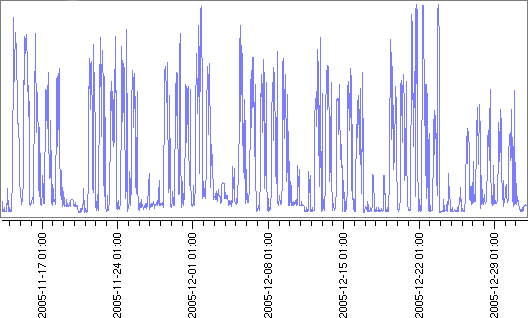In automatic mode, with bi-periodic data, the ARIMA algorithm will only take the long period as main period. For example, in the network traffic case above, using a 1 day period and a 7 day long period would be equivalent to try to find the best ARIMA model with a 7 day period, then find the best neural model with a 1 day period and a 7 day long period, finally keeping the best of the 2.
Another special case would be data suspected as periodic but with a period unknown to the user, in which case, in automatic mode, the period would be set to 0, which the neural algorithm would simply ignore at its turn to fit.

Once the method and the periodic nature of the data are specified, click on the Fit button to start the search for the best model. A progress bar then appears in the State row, successively showing the progress of ARIMA and neural algorithms in automatic mode. You may abort the process at any time by clicking on the Abort button, which replaces the fit button once the fitting process is started.

When the lengthy fitting process is finished, the Residuals graph becomes visible at the bottom of the plot area, while the best method is displayed in the state line, with its parameters in parentheses:

• the ARIMA model may have 3 parameters or 6 if the data was found to be periodic
• the neural model has 2 to 4 parameters depending on the data periodicity

Note that with both models, the bigger the numbers the more complex the model.
Note: for detailed information on the models and their parameters, see the statistics techniques section below.

The residuals graph shows the fitting errors, which allows the user to evaluate the precision of the optimal model found, the smaller the errors the better the fit obviously. You may change the color of the errors graph or hide it, or possibly display it alone for more precision by hiding the original and prepared graphs.

Tip: since preparing the data erases all fitting results and potentially a good found fitted model after a very long fitting process, it is better to use 2 or more predictors on the same data cell, alternately preparing and fitting on different predictors, till at the end, only the predictor with the best results is kept in a saved dashboard.

Again, please do take the time to practice using the sample time series included in the database.

#### 4.3. Predicting future behavior

Once you are satisfied with the fitted model, input a reasonable duration (around 10 to 20 % of the total data time range) to predict the behavior of the prepared data in the future.
Click on the Predict button, and after a short while, the Predicted label should light up and the predicted data graph should become visible, extending the prepared data graph.

#### 4.4. Saving and restoring

Like any viewer, the predictor can be saved and restored as part of a dashboard, including its optimal statistical model once it has been found by the computer.
In all cases, the preparation parameters, the fitting periods, the predict duration, the colors and states of the different graphs are saved and restored.
Only if a best fitted model has been found and saved, is the data automatically prepared and fitted with the saved method and parameters upon restoration. The data is never automatically predicted.

### 5. Statistics techniques

The principle of the fitting process is to try many models for each method (ARIMA and neural) and find the model and its parameters, with the lowest criterion, an approximation of the Schwarz-Bayesian information criterion, defined as follows: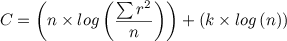with:

• n: number of fitted points
• r: residuals
• k: number of parameters of the model (weights for neural, regression coefficients for ARIMA)

Note that this criterion is a compromise, which imposes a penalty to complex models (with too many parameters).

In the case of the ARIMA method, for the non-seasonal part of the model, the three components (p, d, q) are the AR order, the degree of differencing, and the MA order. For each parameter (p, d and q), the values 0, 1 and 2 will be tried. If the user marked the data as periodic, a seasonal part with (P, D, Q) parameters and the period, will be added to the model, and again all the combinations of 0, 1 and 2 will be tried for the seasonal parameters. The model with the lowest criterion value is then retained.
Once the best ARIMA model is found, provided there is no better neural model in automatic mode, the result is displayed as follows in the fit area state line: ARIMA(p,d,q) or ARIMA(p,d,q,P,D,Q) if the data is periodic.
For more information, please refer to the arima function documentation in the stats R package, and the actual implementation in the predwork.tcl file from the moodss source distribution.

In the case of the neural method, an artificial neural network, of the popular feed-forward type, with a variable number of hidden layers and input neurons, is used. For each number of hidden layers (1, 2 and 3), the network is trained with the prepared values, minus the oldest values corresponding to the specified period or long period if it exists, and the maximum of the lags used (see below). The number of input neurons and the data they are fed depends on the periodicity of the data:

• no period: 1 to 8 input neurons are fed a corresponding number of lagged data samples, making the input data set a window moving from the past to the present of the prepared data
• 1 period: 1 to 6 input neurons are fed data samples, with 2 moving windows separated by the number of points corresponding to the period, the first window with 1 to 3 samples, the last window with 0 to 3 samples
• 2 periods: 1 to 6 input neurons are fed data samples, with 3 moving windows, the 2 first separated by the number of points corresponding to the period, the first and the last separated by the number of points corresponding to the long period, the first window with 1 to 2 samples, the other windows with 0 to 2 samples

The model with the lowest criterion value is then retained. Once the best neural model is found, provided there is no better ARIMA model in automatic mode, the result is displayed as follows in the fit area state line:
• no period: neural(h,l) with h the number of hidden layers and l the size of the moving window
• 1 period: neural(h,l) or neural(h,l,p) with p the size of the last moving window (corresponding to the period)
• 2 periods: neural(h,l) or neural(h,l,p) or neural(h,l,p,P) with P the size of the last moving window (corresponding to the long period)

For more information, please refer to the nnet function documentation in the nnet R package, and the actual implementation in the predwork.tcl file from the moodss source distribution.